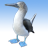# How to format a VB script in Python for Calculate Field?

553
2
06-14-2013 01:17 AMNew Contributor
I have a script for my model in VB which I am running on arcgis 9.3. The same script is for calculate field is not running on arc gis 10.
Do I need to recode or format it in python? I am not good in python so i appreciate if this problem can be solved.
I am mentioning the syntax of vb script for reference.

gp.CalculateField_management(New_shp__2_, "Angle", "pp", "VB", "dim pp as double\\nif [dir_y]>0 and [dir_x] > 0 then \\npp=57.27 *ATN([dir_y]/[dir_x])\\nelseif [dir_y]>0 and [dir_x]<0 then \\npp=180-(57.27*ATN(-[dir_y]/[dir_x]))\\nelseif [dir_y]>0 and [dir_x] = 0  then\\npp=90\\n\\nelseif [dir_y]<0 and [dir_x]>0 then \\npp=360 - ABS(57.27*(-ATN(-[dir_y]/[dir_x])))\\nelseif [dir_y]<0 and [dir_x]<0 then \\npp= 360-ABS(57.27*ATN([dir_y]/[dir_x])-180)\\nelseif [dir_y]<0 and [dir_x]=0 then \\npp=270\\n\\n\\nelseif [dir_y]<0     and [dir_x]>0 then \\npp=360 - ABS(57.27*(-ATN(-[dir_y]/[dir_x])))\\nelseif [dir_y]<0 and [dir_x]<0 then \\npp= 360 - ABS (57.27 * ATN([dir_y]/[dir_x])-180)\\nelseif [dir_y]<0 and [dir_x]=0 then \\npp=270\\n\\n\\nelseif [dir_x] > 0 then\\npp=0\\nelseif [dir_x] > 0   and [dir_x]<0 then\\npp=180\\nelse\\npp=0\\nend if\\n")
Tags (3)
2 RepliesFrequent Contributor
Use still specify the code as 'VB', that is good, but it's really VBScript...

"VBScript does not allow you to explicitly declare any data types; all variables are implicitly Variant. Statements like Dim x as String should be removed or simplified to Dim x."

http://help.arcgis.com/en/arcgisdesktop/10.0/help/index.html#/Calculate_Field_examples/00170000004s0...New Contributor
Thank you So much sir... I made changes u suggested and it is running in arc 10...
but this syntax is running on arc 9.3..
thanks for the quick reply and help :):)